Скачать презентацию Chapter 5 Bond Prices and Interest Rate Risk

5e029bc2286babe1cf216f04aac886ec.ppt

• Количество слайдов: 31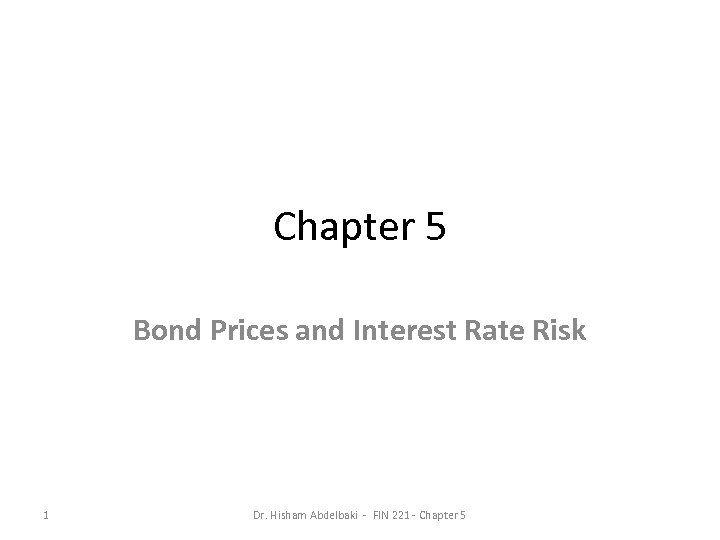Chapter 5 Bond Prices and Interest Rate Risk 1 Dr. Hisham Abdelbaki - FIN 221 - Chapter 5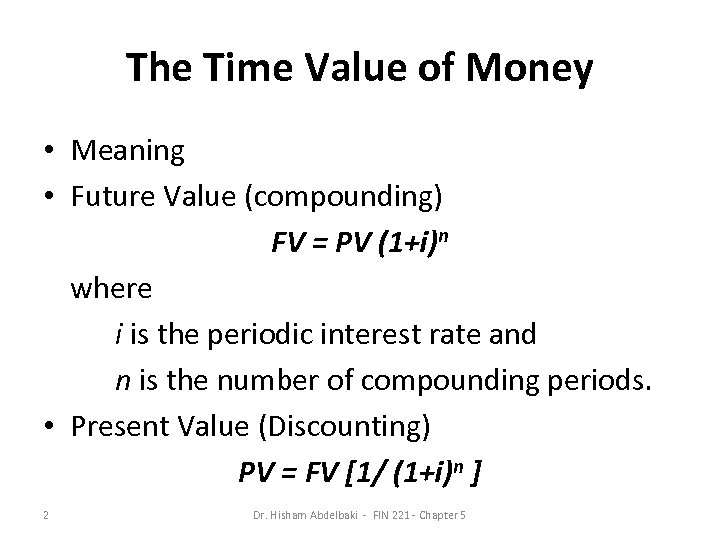The Time Value of Money • Meaning • Future Value (compounding) FV = PV (1+i)n where i is the periodic interest rate and n is the number of compounding periods. • Present Value (Discounting) PV = FV [1/ (1+i)n ] 2 Dr. Hisham Abdelbaki - FIN 221 - Chapter 5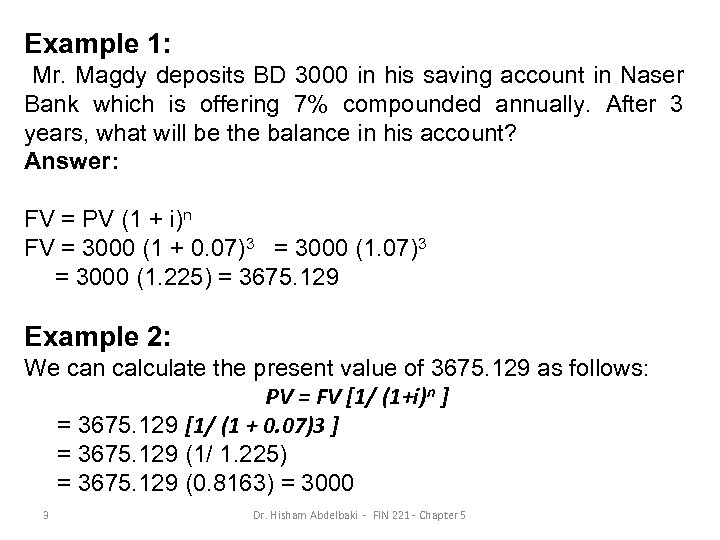Example 1: Mr. Magdy deposits BD 3000 in his saving account in Naser Bank which is offering 7% compounded annually. After 3 years, what will be the balance in his account? Answer: FV = PV (1 + i)n FV = 3000 (1 + 0. 07)3 = 3000 (1. 225) = 3675. 129 Example 2: We can calculate the present value of 3675. 129 as follows: PV = FV [1/ (1+i)n ] = 3675. 129 [1/ (1 + 0. 07)3 ] = 3675. 129 (1/ 1. 225) = 3675. 129 (0. 8163) = 3000 3 Dr. Hisham Abdelbaki - FIN 221 - Chapter 5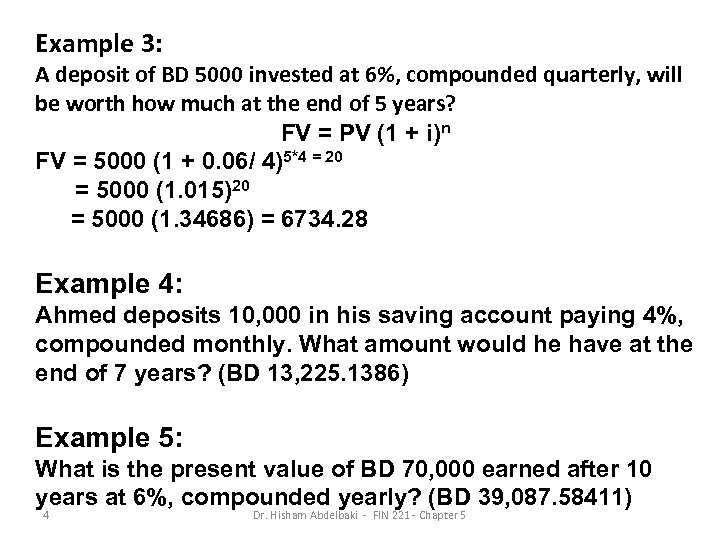Example 3: A deposit of BD 5000 invested at 6%, compounded quarterly, will be worth how much at the end of 5 years? FV = PV (1 + i)n FV = 5000 (1 + 0. 06/ 4)5*4 = 20 = 5000 (1. 015)20 = 5000 (1. 34686) = 6734. 28 Example 4: Ahmed deposits 10, 000 in his saving account paying 4%, compounded monthly. What amount would he have at the end of 7 years? (BD 13, 225. 1386) Example 5: What is the present value of BD 70, 000 earned after 10 years at 6%, compounded yearly? (BD 39, 087. 58411) 4 Dr. Hisham Abdelbaki - FIN 221 - Chapter 5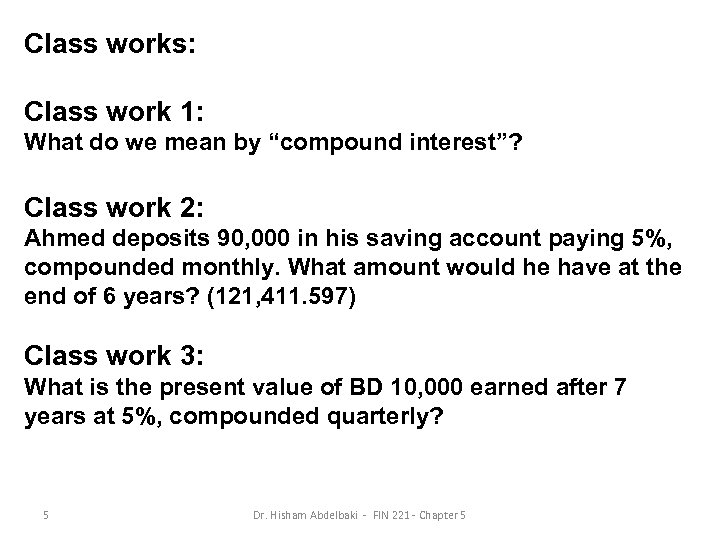Class works: Class work 1: What do we mean by “compound interest”? Class work 2: Ahmed deposits 90, 000 in his saving account paying 5%, compounded monthly. What amount would he have at the end of 6 years? (121, 411. 597) Class work 3: What is the present value of BD 10, 000 earned after 7 years at 5%, compounded quarterly? 5 Dr. Hisham Abdelbaki - FIN 221 - Chapter 5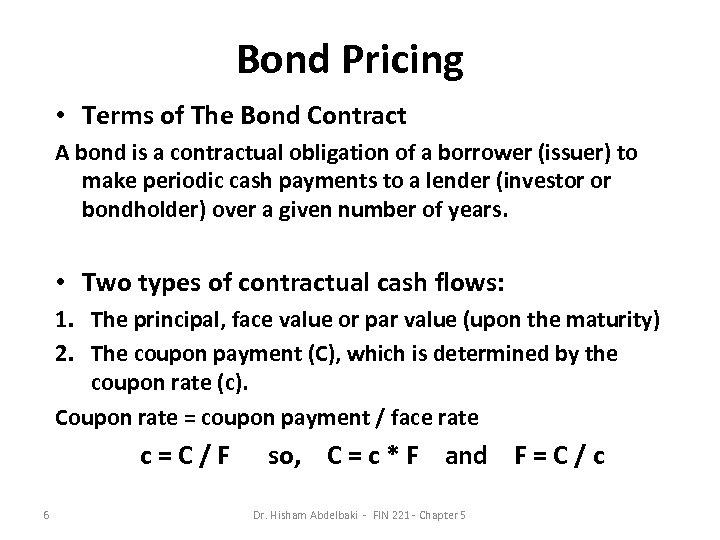Bond Pricing • Terms of The Bond Contract A bond is a contractual obligation of a borrower (issuer) to make periodic cash payments to a lender (investor or bondholder) over a given number of years. • Two types of contractual cash flows: 1. The principal, face value or par value (upon the maturity) 2. The coupon payment (C), which is determined by the coupon rate (c). Coupon rate = coupon payment / face rate c=C/F 6 so, C = c * F and F = C / c Dr. Hisham Abdelbaki - FIN 221 - Chapter 5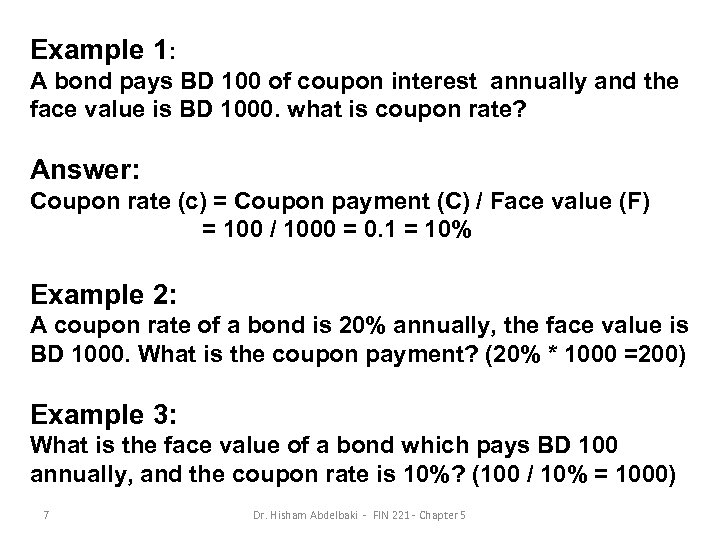Example 1: A bond pays BD 100 of coupon interest annually and the face value is BD 1000. what is coupon rate? Answer: Coupon rate (c) = Coupon payment (C) / Face value (F) = 100 / 1000 = 0. 1 = 10% Example 2: A coupon rate of a bond is 20% annually, the face value is BD 1000. What is the coupon payment? (20% * 1000 =200) Example 3: What is the face value of a bond which pays BD 100 annually, and the coupon rate is 10%? (100 / 10% = 1000) 7 Dr. Hisham Abdelbaki - FIN 221 - Chapter 5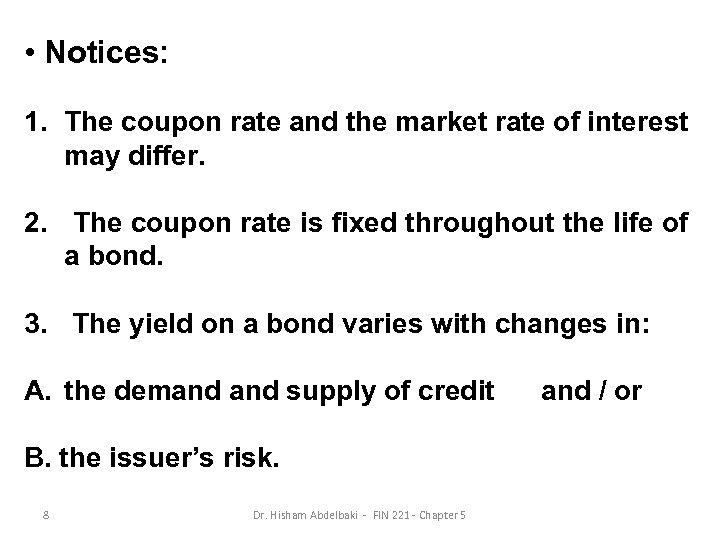• Notices: 1. The coupon rate and the market rate of interest may differ. 2. The coupon rate is fixed throughout the life of a bond. 3. The yield on a bond varies with changes in: A. the demand supply of credit B. the issuer’s risk. 8 Dr. Hisham Abdelbaki - FIN 221 - Chapter 5 and / or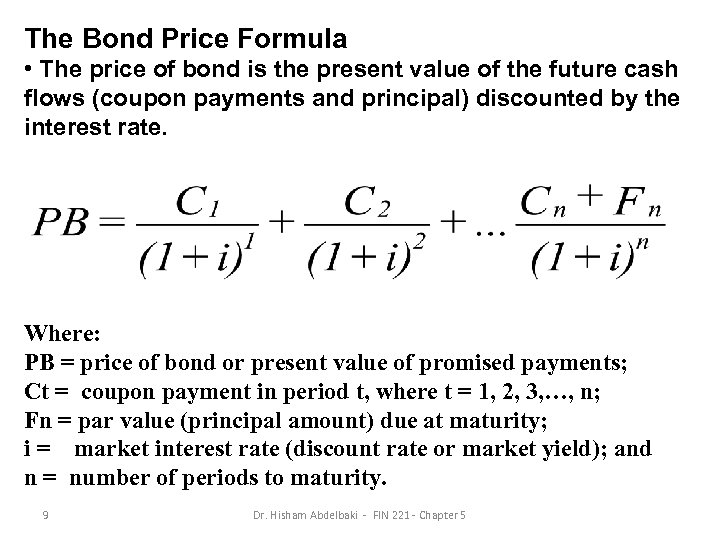The Bond Price Formula • The price of bond is the present value of the future cash flows (coupon payments and principal) discounted by the interest rate. Where: PB = price of bond or present value of promised payments; Ct = coupon payment in period t, where t = 1, 2, 3, …, n; Fn = par value (principal amount) due at maturity; i = market interest rate (discount rate or market yield); and n = number of periods to maturity. 9 Dr. Hisham Abdelbaki - FIN 221 - Chapter 5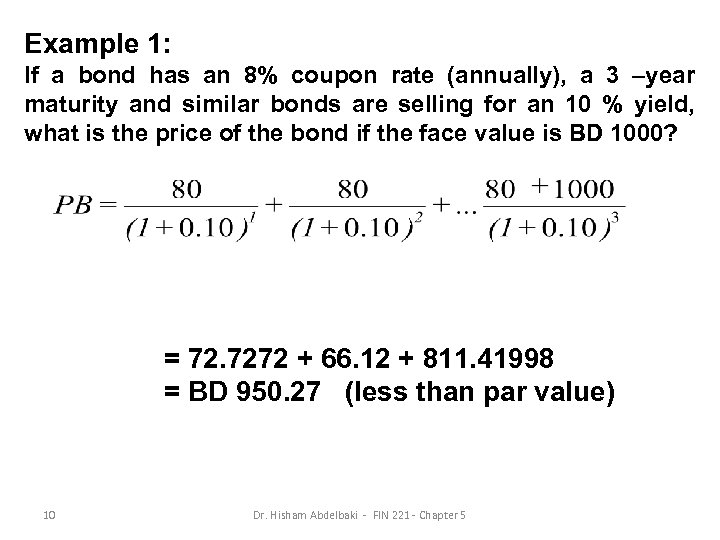Example 1: If a bond has an 8% coupon rate (annually), a 3 –year maturity and similar bonds are selling for an 10 % yield, what is the price of the bond if the face value is BD 1000? = 72. 7272 + 66. 12 + 811. 41998 = BD 950. 27 (less than par value) 10 Dr. Hisham Abdelbaki - FIN 221 - Chapter 5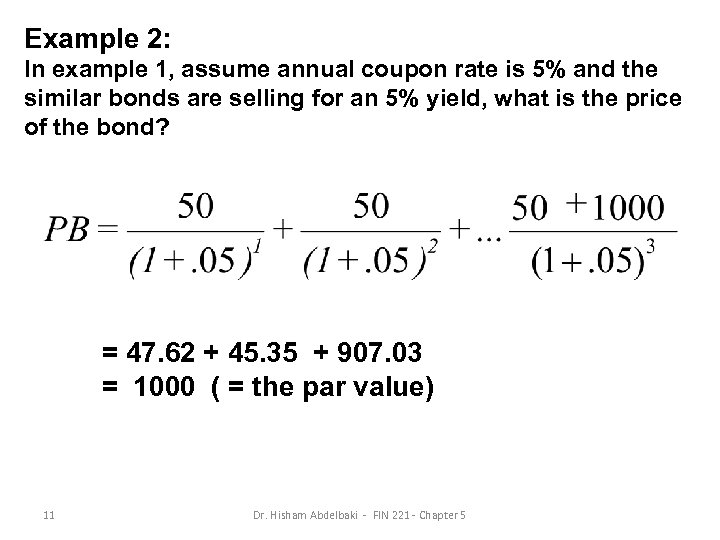Example 2: In example 1, assume annual coupon rate is 5% and the similar bonds are selling for an 5% yield, what is the price of the bond? = 47. 62 + 45. 35 + 907. 03 = 1000 ( = the par value) 11 Dr. Hisham Abdelbaki - FIN 221 - Chapter 5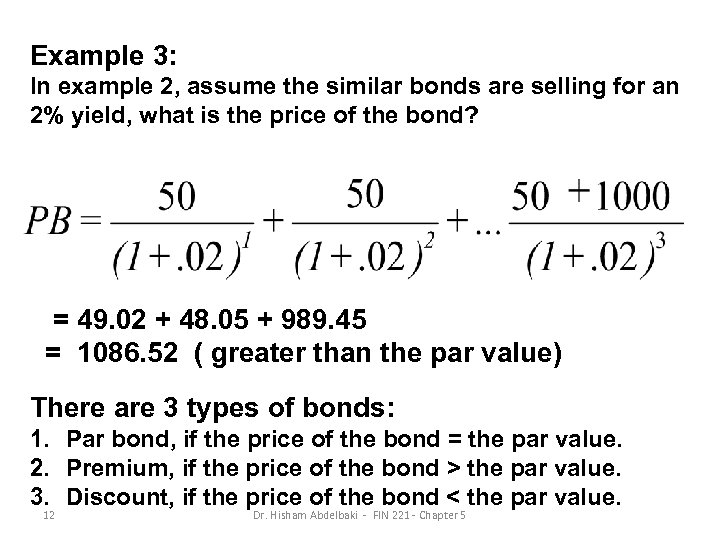Example 3: In example 2, assume the similar bonds are selling for an 2% yield, what is the price of the bond? = 49. 02 + 48. 05 + 989. 45 = 1086. 52 ( greater than the par value) There are 3 types of bonds: 1. Par bond, if the price of the bond = the par value. 2. Premium, if the price of the bond > the par value. 3. Discount, if the price of the bond < the par value. 12 Dr. Hisham Abdelbaki - FIN 221 - Chapter 5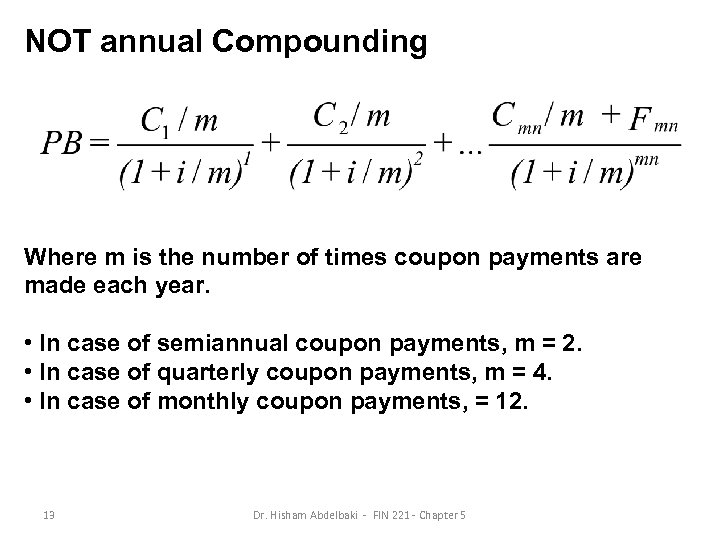NOT annual Compounding Where m is the number of times coupon payments are made each year. • In case of semiannual coupon payments, m = 2. • In case of quarterly coupon payments, m = 4. • In case of monthly coupon payments, = 12. 13 Dr. Hisham Abdelbaki - FIN 221 - Chapter 5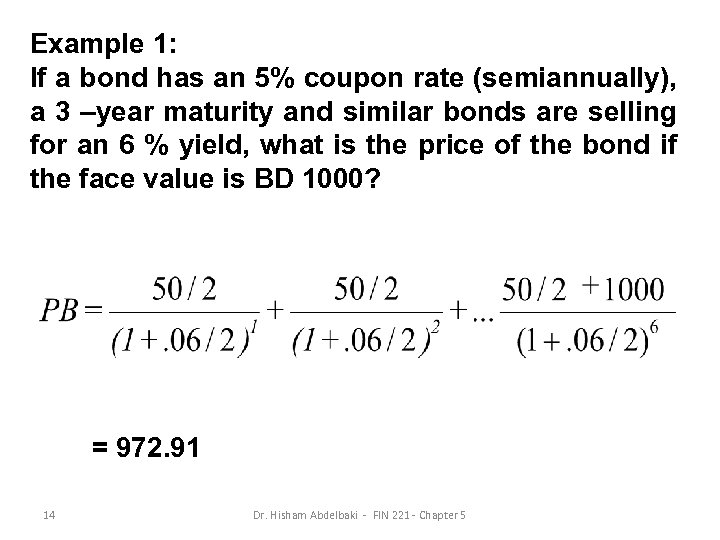Example 1: If a bond has an 5% coupon rate (semiannually), a 3 –year maturity and similar bonds are selling for an 6 % yield, what is the price of the bond if the face value is BD 1000? = 972. 91 14 Dr. Hisham Abdelbaki - FIN 221 - Chapter 5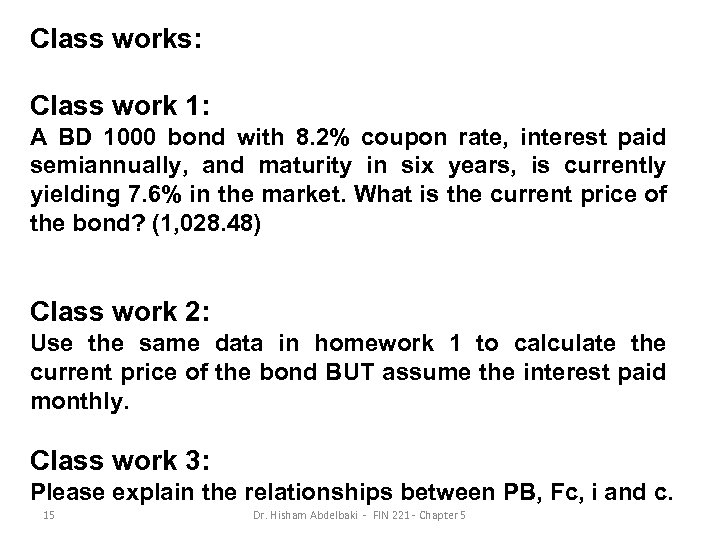Class works: Class work 1: A BD 1000 bond with 8. 2% coupon rate, interest paid semiannually, and maturity in six years, is currently yielding 7. 6% in the market. What is the current price of the bond? (1, 028. 48) Class work 2: Use the same data in homework 1 to calculate the current price of the bond BUT assume the interest paid monthly. Class work 3: Please explain the relationships between PB, Fc, i and c. 15 Dr. Hisham Abdelbaki - FIN 221 - Chapter 5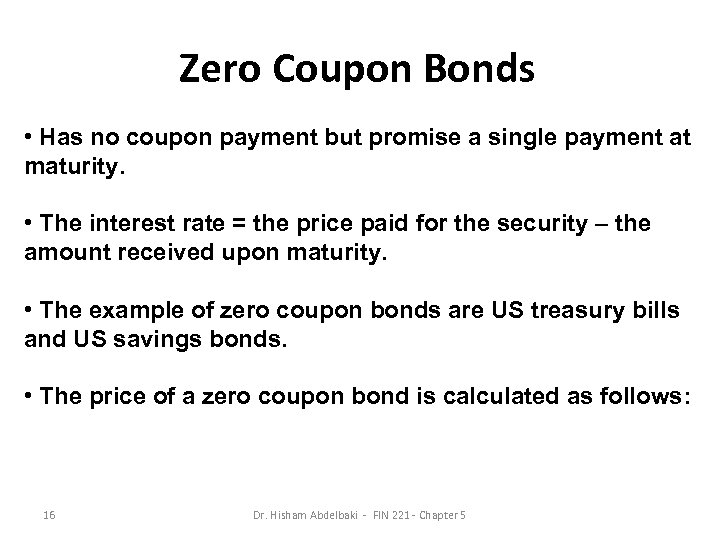Zero Coupon Bonds • Has no coupon payment but promise a single payment at maturity. • The interest rate = the price paid for the security – the amount received upon maturity. • The example of zero coupon bonds are US treasury bills and US savings bonds. • The price of a zero coupon bond is calculated as follows: 16 Dr. Hisham Abdelbaki - FIN 221 - Chapter 5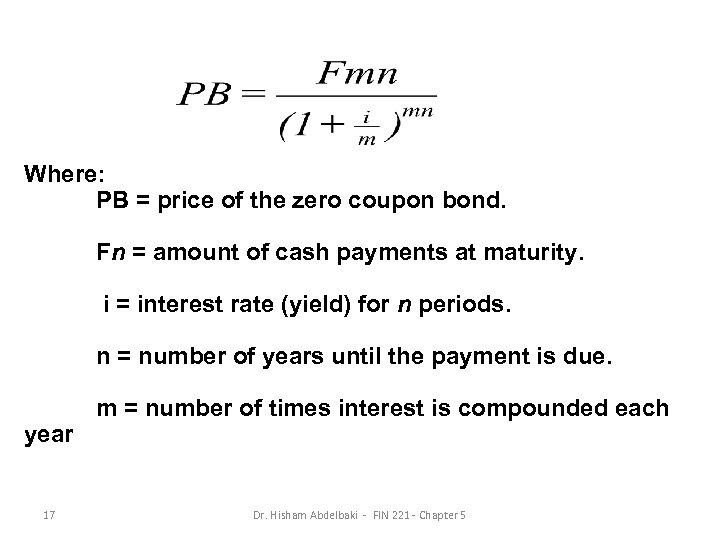Where: PB = price of the zero coupon bond. Fn = amount of cash payments at maturity. i = interest rate (yield) for n periods. n = number of years until the payment is due. year 17 m = number of times interest is compounded each Dr. Hisham Abdelbaki - FIN 221 - Chapter 5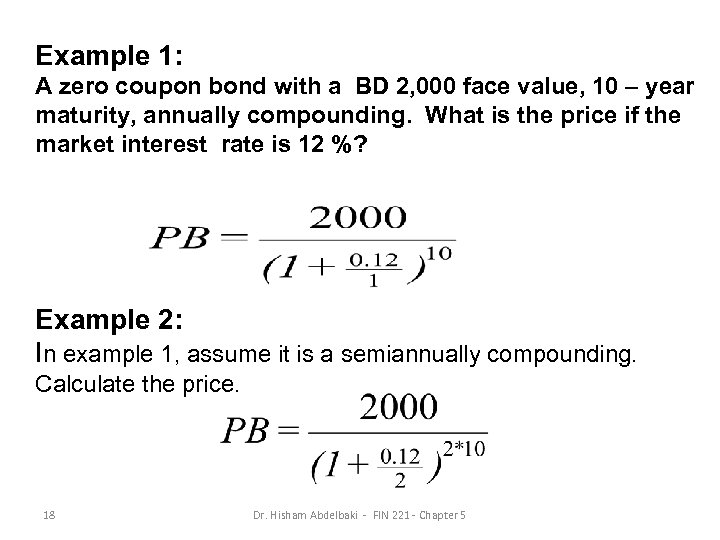Example 1: A zero coupon bond with a BD 2, 000 face value, 10 – year maturity, annually compounding. What is the price if the market interest rate is 12 %? Example 2: In example 1, assume it is a semiannually compounding. Calculate the price. 18 Dr. Hisham Abdelbaki - FIN 221 - Chapter 5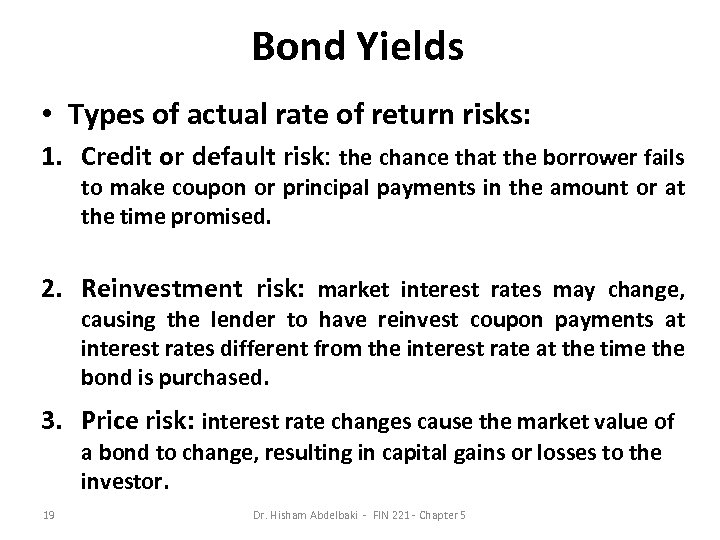Bond Yields • Types of actual rate of return risks: 1. Credit or default risk: the chance that the borrower fails to make coupon or principal payments in the amount or at the time promised. 2. Reinvestment risk: market interest rates may change, causing the lender to have reinvest coupon payments at interest rates different from the interest rate at the time the bond is purchased. 3. Price risk: interest rate changes cause the market value of a bond to change, resulting in capital gains or losses to the investor. 19 Dr. Hisham Abdelbaki - FIN 221 - Chapter 5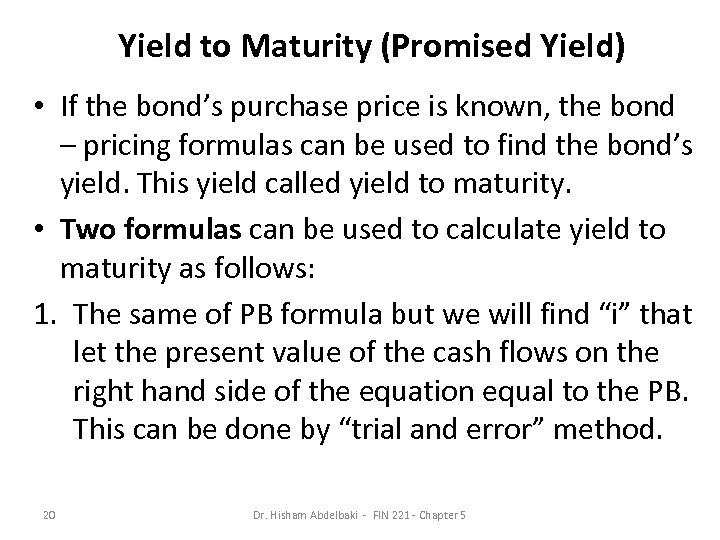Yield to Maturity (Promised Yield) • If the bond’s purchase price is known, the bond – pricing formulas can be used to find the bond’s yield. This yield called yield to maturity. • Two formulas can be used to calculate yield to maturity as follows: 1. The same of PB formula but we will find “i” that let the present value of the cash flows on the right hand side of the equation equal to the PB. This can be done by “trial and error” method. 20 Dr. Hisham Abdelbaki - FIN 221 - Chapter 5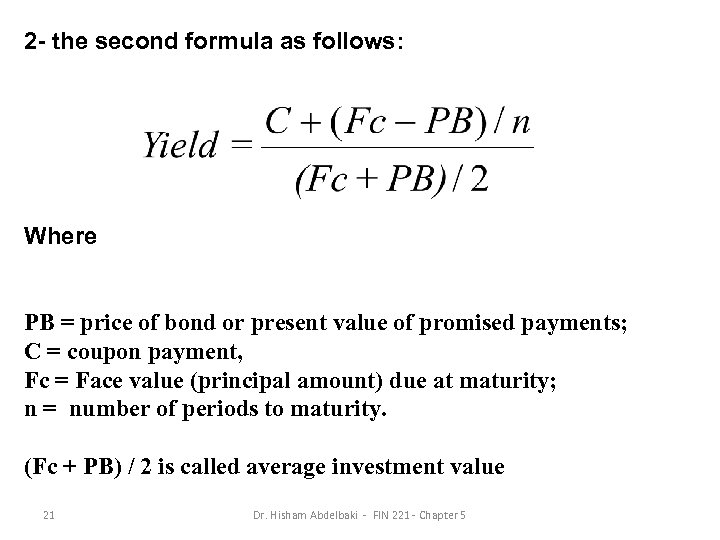2 - the second formula as follows: Where PB = price of bond or present value of promised payments; C = coupon payment, Fc = Face value (principal amount) due at maturity; n = number of periods to maturity. (Fc + PB) / 2 is called average investment value 21 Dr. Hisham Abdelbaki - FIN 221 - Chapter 5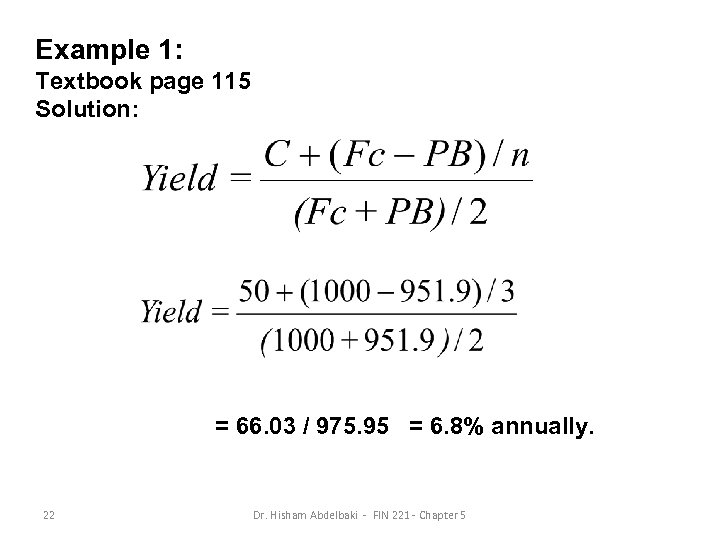Example 1: Textbook page 115 Solution: = 66. 03 / 975. 95 = 6. 8% annually. 22 Dr. Hisham Abdelbaki - FIN 221 - Chapter 5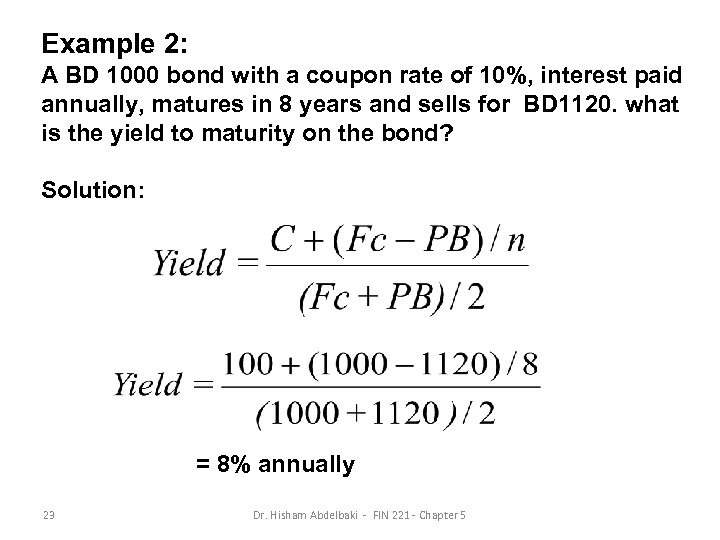Example 2: A BD 1000 bond with a coupon rate of 10%, interest paid annually, matures in 8 years and sells for BD 1120. what is the yield to maturity on the bond? Solution: = 8% annually 23 Dr. Hisham Abdelbaki - FIN 221 - Chapter 5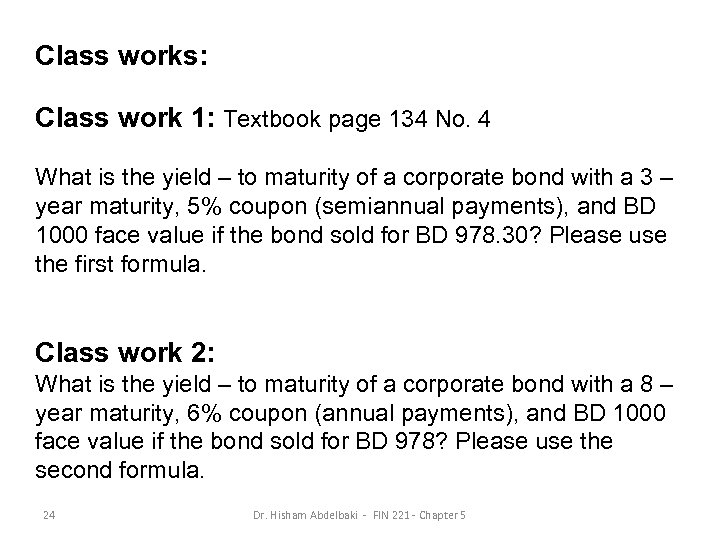Class works: Class work 1: Textbook page 134 No. 4 What is the yield – to maturity of a corporate bond with a 3 – year maturity, 5% coupon (semiannual payments), and BD 1000 face value if the bond sold for BD 978. 30? Please use the first formula. Class work 2: What is the yield – to maturity of a corporate bond with a 8 – year maturity, 6% coupon (annual payments), and BD 1000 face value if the bond sold for BD 978? Please use the second formula. 24 Dr. Hisham Abdelbaki - FIN 221 - Chapter 5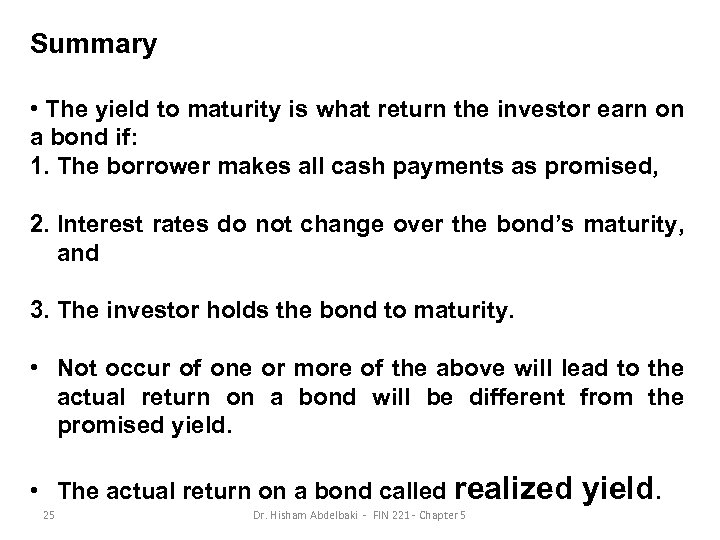Summary • The yield to maturity is what return the investor earn on a bond if: 1. The borrower makes all cash payments as promised, 2. Interest rates do not change over the bond’s maturity, and 3. The investor holds the bond to maturity. • Not occur of one or more of the above will lead to the actual return on a bond will be different from the promised yield. • The actual return on a bond called realized 25 Dr. Hisham Abdelbaki - FIN 221 - Chapter 5 yield.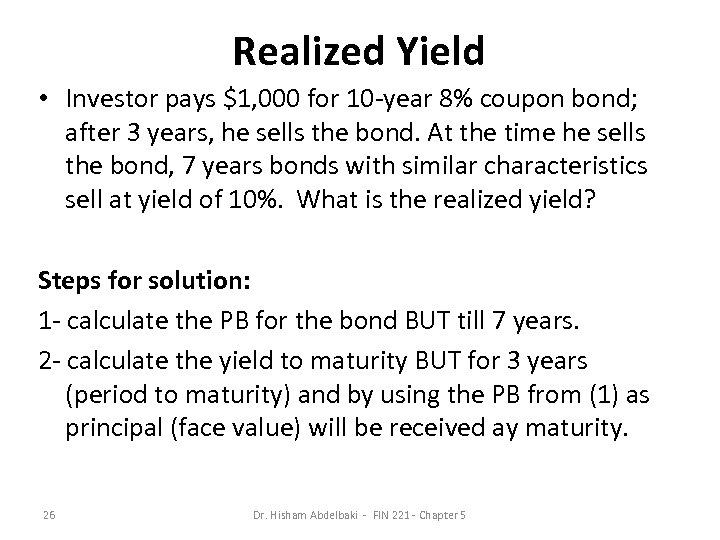Realized Yield • Investor pays \$1, 000 for 10 -year 8% coupon bond; after 3 years, he sells the bond. At the time he sells the bond, 7 years bonds with similar characteristics sell at yield of 10%. What is the realized yield? Steps for solution: 1 - calculate the PB for the bond BUT till 7 years. 2 - calculate the yield to maturity BUT for 3 years (period to maturity) and by using the PB from (1) as principal (face value) will be received ay maturity. 26 Dr. Hisham Abdelbaki - FIN 221 - Chapter 5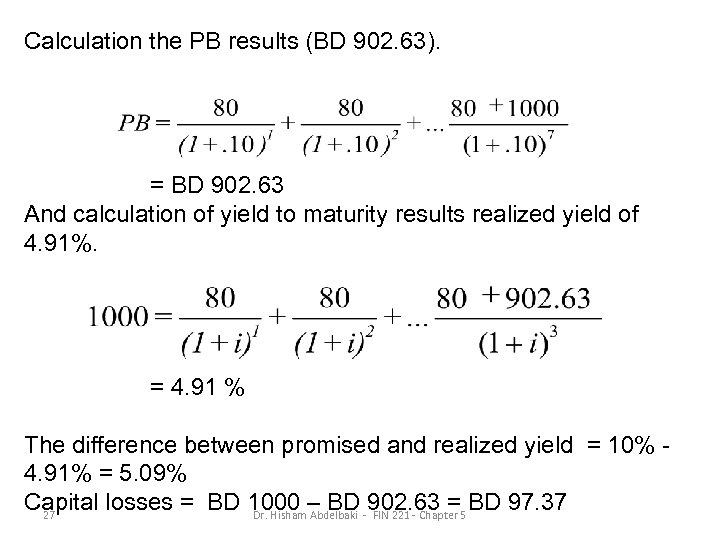Calculation the PB results (BD 902. 63). = BD 902. 63 And calculation of yield to maturity results realized yield of 4. 91%. = 4. 91 % The difference between promised and realized yield = 10% 4. 91% = 5. 09% Capital losses = BD 1000 – BD -902. 63 =5 BD 97. 37 27 Dr. Hisham Abdelbaki FIN 221 - Chapter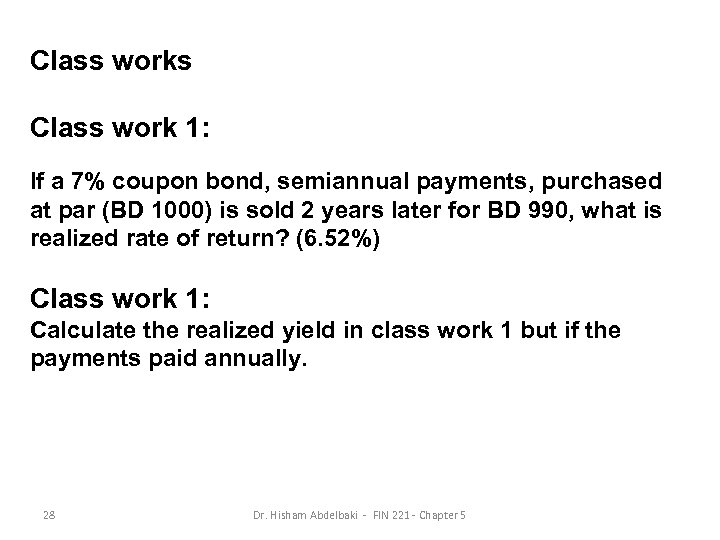Class works Class work 1: If a 7% coupon bond, semiannual payments, purchased at par (BD 1000) is sold 2 years later for BD 990, what is realized rate of return? (6. 52%) Class work 1: Calculate the realized yield in class work 1 but if the payments paid annually. 28 Dr. Hisham Abdelbaki - FIN 221 - Chapter 5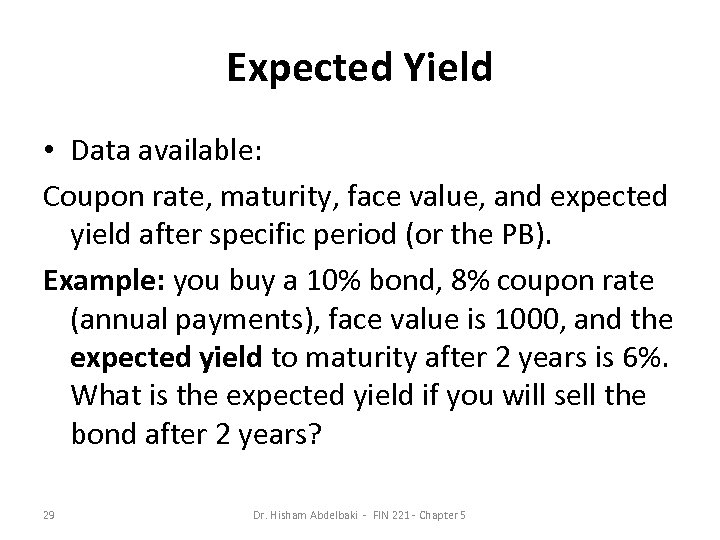Expected Yield • Data available: Coupon rate, maturity, face value, and expected yield after specific period (or the PB). Example: you buy a 10% bond, 8% coupon rate (annual payments), face value is 1000, and the expected yield to maturity after 2 years is 6%. What is the expected yield if you will sell the bond after 2 years? 29 Dr. Hisham Abdelbaki - FIN 221 - Chapter 5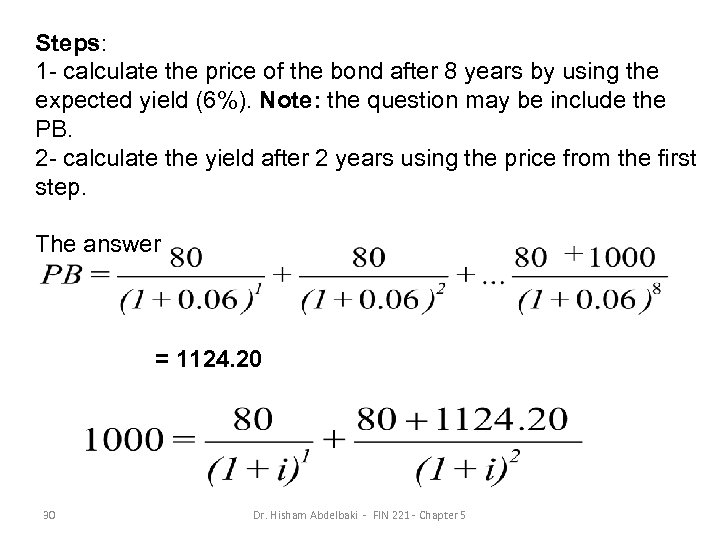Steps: 1 - calculate the price of the bond after 8 years by using the expected yield (6%). Note: the question may be include the PB. 2 - calculate the yield after 2 years using the price from the first step. The answer = 1124. 20 30 Dr. Hisham Abdelbaki - FIN 221 - Chapter 5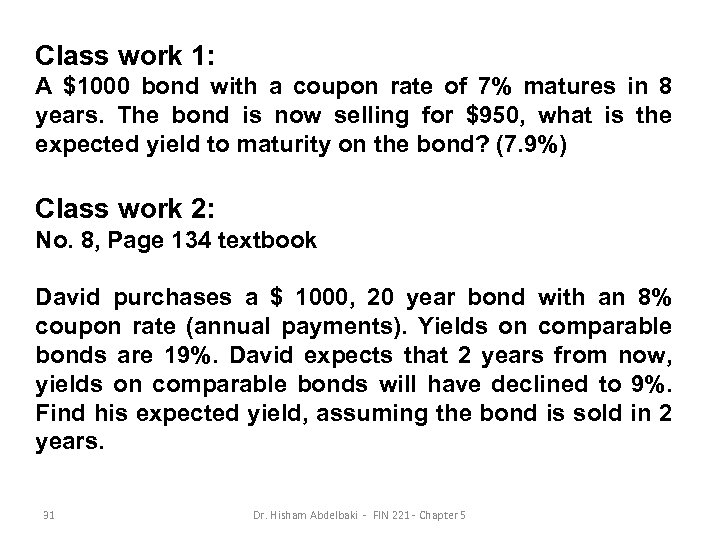Class work 1: A \$1000 bond with a coupon rate of 7% matures in 8 years. The bond is now selling for \$950, what is the expected yield to maturity on the bond? (7. 9%) Class work 2: No. 8, Page 134 textbook David purchases a \$ 1000, 20 year bond with an 8% coupon rate (annual payments). Yields on comparable bonds are 19%. David expects that 2 years from now, yields on comparable bonds will have declined to 9%. Find his expected yield, assuming the bond is sold in 2 years. 31 Dr. Hisham Abdelbaki - FIN 221 - Chapter 5